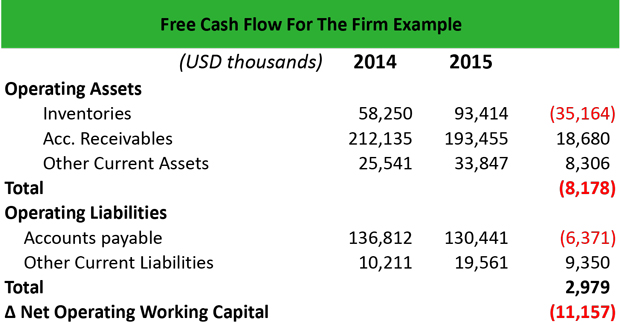# What is Free Cash Flow For The Firm (FCFF)?

Definition: The free cash flow for the firm (FCFF) represents the amount of cash that is available for distribution to shareholders after working capital, capital expenditure, taxes and depreciation expenses are paid.

## What Does Free Cash Flow For the Firm Mean?

What is the definition of free cash flow for the firm? The FCFF is a performance valuation metric that financial analysts use as a benchmark to analyze a firm’s financial health. Once a company has paid the short-term and long-term obligations, including salaries, the cost of goods sold, R&D, depreciation, taxes, and reinvestments in the business, the amount that is left is the FCFF.

To calculate FCFF, we need to know EBIT, the tax rate, depreciation, capital expenditures (CAPEX), and changes in working capital.Let’s look at an example.

## Example

Mark is a financial analyst in a brokerage firm. He is asked to calculate the FCFF of a retail company that trades on the NYSE and compare it to a few similar companies as well as to its own past performance.

Mark creates an Excel spreadsheet, and he calculates first the changes in the firm’s working capital:

In the financial statements of 2015, the retail company reports EBIT \$125,000, depreciation \$23,500, and CapEx \$98,200. The tax rate is 35%. Mark calculates the free cash flow for the firm as follows:

FCFF = EBIT (1-t) + Depreciation – CapEx – change in NOWC = 125,000 x (1-35%) + 23,500 – 98,200 – (-11,157) = 81,250 + 23,500 – 98,200 + 11,157 = \$17,707.

Therefore, the FCFF is \$17.7 million and is available to shareholders. For a financial analysis perspective, FCFF is important when valuing a stock because it offers an indication of a firm’s future cash flows expressed in today’s values.

## Summary Definition

Define Does Free Cash Flow For the Firm: FCFF means a profitability calculation that measures the amount of money left over for shareholders after all operating expenses have been paid.